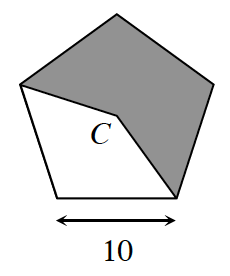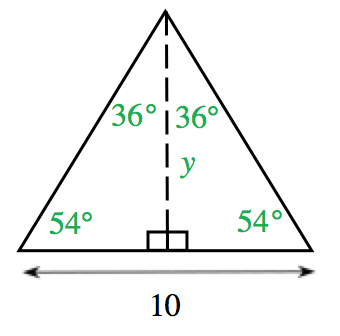### Home > INT2 > Chapter 9 > Lesson 9.3.3 > Problem9-110

9-110.What is the area of the shaded region for the regular pentagon at right if the length of each side of the pentagon is $10$ units? Assume that point $C$ is the center of the pentagon.

A regular pentagon is made up of $5$ isosceles triangles with angle measures $72°$, $54°$, and $54°$.

First find the area of one of the five triangles within the pentagon, like the one shown at right.$\text{tan}(54°)=\frac{y}{5}$

$y = 5\tan(54°)$

$y ≈ 6.88$

Area $= .5$(base)(height) $= .5(10)(6.88) ≈ 34.41$

The area of the shaded region is equal to three of these triangles, so $3(34.41) ≈ 103.23$ units